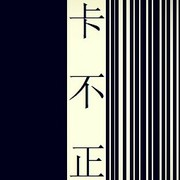[C]_ _ _ 很想 知道你[Am]近况 我听人[F]说 还不如[G]你对我讲　 [C]_ _ _ 经过 那段[Am]遗憾 请你[F]放 心 我[G]变得更加坚强 [F]　世界[C]不管怎样[Dm]荒凉 [G]　 [F]　爱过[C]你就不怕[Dm]孤单 [G] {start_of_chorus} 我最[C]亲爱的 你过的怎么[Am]样 没我[F]的日子 你别来无[G]恙 依然[C]亲爱的 我没让你失[Am]望 让我[F]亲一亲 像过去一[G]样 {end_of_chorus} [C]_ _ _ 我想你 一定[Am]喜欢 现在的[F]我 学会了[G]你最爱的开朗 [C]_ _ _ 想起 你的[Am]模样 有什[F]么 错 还[G]不能够被原谅 [F]　世界[C]不管怎样[Dm]荒凉 [G]　 [F]　爱过[C]你就不怕[Dm]孤单 [G]　 {start_of_chorus} 我最[C]亲爱的 你过的怎么[Am]样 没我[F]的日子 你别来无[G]恙 依然[C]亲爱的 我没让你失[Am]望 让我[F]亲一亲 像朋友一[G]样 {end_of_chorus} [C] 虽然离开了你的[Am]时间 比一起[F]还漫长 我[G]们总能补偿 [C] 因为中间空白的[Am]时光 如果还[F]能 分享 也[G]是一种浪漫 [F]　关系[C]虽然不再[Dm]一样 [G]　 [F]　关心[C]却怎么能[Dm]说断 [Dm] 就[G]断 [G] {start_of_chorus} 我最[C]亲爱的 你过的怎么[Am]样 没我[F]的日子 你别来无[G]恙 依然[C]亲爱的 我没让你失[Am]望 让我[F]亲一亲 像亲人一[G]样 {end_of_chorus} {start_of_chorus} 我最[C]亲爱的 你过的怎么[Am]样 没我[F]的日子 你别来无[G]恙 依然[C]亲爱的 我没让你失[Am]望 让我[F]亲一亲 像过去一[G]样 {end_of_chorus} {title:我最亲爱的} {artist:张惠妹} {author:_wc_wc_}_wc_wc_